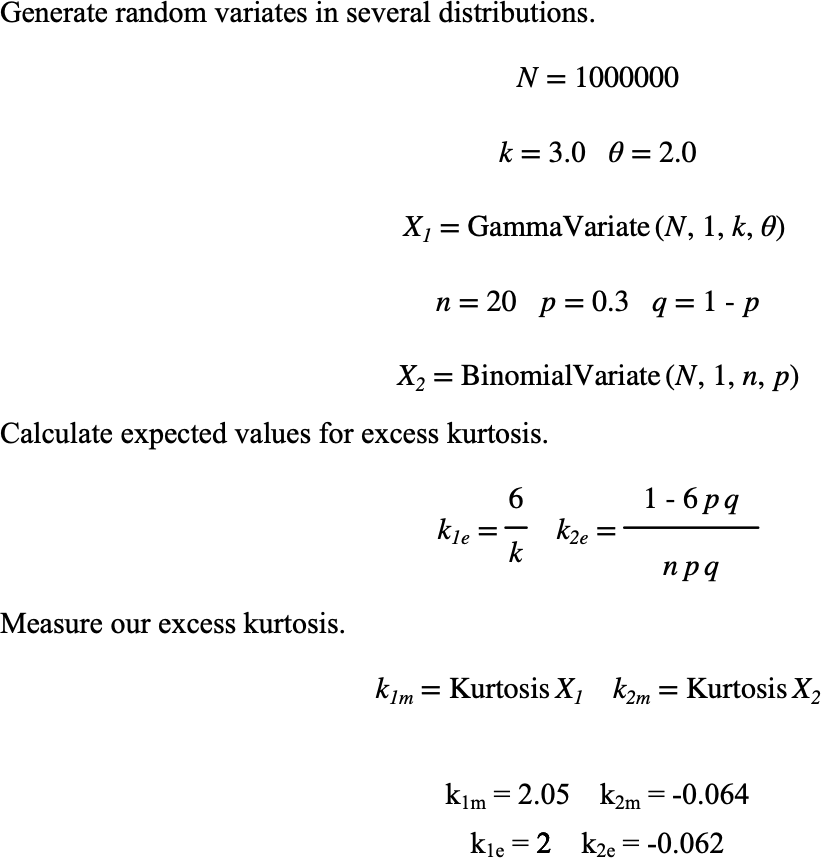# $$\text{Kurtosis}$$¶

You can use the $$\text{Kurtosis}$$ function to calculate the excess Kurtosis of a collection of values.

You can use the \kurtosis backslash command to insert this function.

The following variants of this function are available:

• $$\text{real } \text{Kurtosis} \left ( \ldots \right )$$

Note that this function works for all data types and, like the $$\text{Average}$$ and $$\text{Count}$$ functions can recursively traverse iterable types to locate scalar values to include in the excess kurtosis calculation.

The excess kurtosis is calculated by:

$\text{Kurtosis} \left ( X \right ) = \left [ \frac{1}{N} \sum_{ x \in X } \left ( \frac{ x - \mu }{\sigma} \right ) ^ 4 \right ] - 3$

Figure 163 shows the basic use of the $$\text{Kurtosis}$$ function.Figure 163 Example Use Of The Kurtosis Function